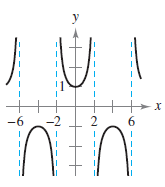×
Get Full Access to Calculus: Early Transcendental Functions - 6 Edition - Chapter 2.5 - Problem 4
Get Full Access to Calculus: Early Transcendental Functions - 6 Edition - Chapter 2.5 - Problem 4

×

# ?Determining Infinite Limits from a Graph In Exercises 1-4, determine whether f(x) approaches $$\infty$$ or $$-\infty$$ as x approaches -2 from the lefISBN: 9781285774770 141

## Solution for problem 4 Chapter 2.5

Calculus: Early Transcendental Functions | 6th Edition

• Textbook Solutions
• 2901 Step-by-step solutions solved by professors and subject experts
• Get 24/7 help from StudySoup virtual teaching assistantsCalculus: Early Transcendental Functions | 6th Edition

4 5 1 416 Reviews
12
2
Problem 4

Determining Infinite Limits from a Graph In Exercises 1-4, determine whether f(x) approaches $$\infty$$ or $$-\infty$$ as x approaches -2 from the left and from the right.

$$f(x)=\sec \frac{\pi x}{4}$$Text Transcription:

infinity

-infinity

f(x)=sec pi x/4

Step-by-Step Solution:

Step 1 of 5) Finding a Binomial Series Find the power series for Solution Using the binomial series

Step 2 of 2

##### ISBN: 9781285774770

The answer to “?Determining Infinite Limits from a Graph In Exercises 1-4, determine whether f(x) approaches $$\infty$$ or $$-\infty$$ as x approaches -2 from the left and from the right.$$f(x)=\sec \frac{\pi x}{4}$$ Text Transcription:infinity-infinityf(x)=sec pi x/4” is broken down into a number of easy to follow steps, and 33 words. This full solution covers the following key subjects: . This expansive textbook survival guide covers 134 chapters, and 10738 solutions. This textbook survival guide was created for the textbook: Calculus: Early Transcendental Functions, edition: 6. Calculus: Early Transcendental Functions was written by and is associated to the ISBN: 9781285774770. The full step-by-step solution to problem: 4 from chapter: 2.5 was answered by , our top Calculus solution expert on 11/14/17, 10:53PM. Since the solution to 4 from 2.5 chapter was answered, more than 249 students have viewed the full step-by-step answer.

## Discover and learn what students are asking

Calculus: Early Transcendental Functions : Basic Differentiation Rules and Rates of Change
?How Do You See It? Use the graph of f to answer each question. To print an enlarged copy of the graph, go to MathGraphs.com. (a) Betwee

Statistics: Informed Decisions Using Data : Inference about Two Population Standard Deviations
?In Problems 9–14, assume that the populations are normally distributed. Test the given hypothesis. 12. s1 6 s2 at the a = 0.05 level of signi

Unlock Textbook Solution

?Determining Infinite Limits from a Graph In Exercises 1-4, determine whether f(x) approaches $$\infty$$ or $$-\infty$$ as x approaches -2 from the lef